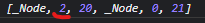# Get nodeBefore for current cursor position if `depth=1`

Hi, I have the following structure.

I want to select `Math!` node when pressing backspace at the beginning of `Paragraph 2`. I tried to use `\$from.nodeBefore` but it return null because there is no node before the current depth (1). How to get this node? `path` shows:so the current node is the node with index `2` in the `doc` node. How to get this `2` from resolved positioned so that I could pick no at index `1` (`doc.child(1)`) from `doc` which is the desired math node?

Check that `\$from.index(-1)` is > 0 and then take `\$from.node(-1).child(\$from.index(-1))`. Or even loop up the tree from `\$from` (looping depth from `\$from.depth` to 0) to check whether there is a math node right in front of the position (and stopping if there’s other stuff before it at any level).

1 Like

Shouldn’t it be `\$from.node(-1).child(\$from.index(-1) - 1);`? Because `\$from.node(-1).child(\$from.index(-1))` returns the same node. I understand it in such a way that `\$from.node(-1)` returns the parent node (i.e. `doc`) and then using `child(\$from.index(-1) - 1)` we can get the previous child node. Before you replied I did the following:

``````    let index = \$from.index(\$from.depth - 1);
let pos = \$from.posAtIndex(index - 1, \$from.depth - 1);
let node = state.tr.doc.nodeAt(pos);
if (
node?.type.name === "math_display" &&
pos + node?.nodeSize! + 1 === \$from.pos
) {
view.dispatch(
state.tr.setSelection(NodeSelection.create(state.tr.doc, pos))
);
return true;
}
return false;

``````

Are these solutions equivalent and is my code correct?

Yes it should.

Note that your code won’t work if there’s, for example, a list wrapping the block with the cursor, because then you’d need to go up multiple levels.